# Describing Motion of a Rigid Body (Part - 1) Civil Engineering (CE) Notes | EduRev

## Civil Engineering (CE) : Describing Motion of a Rigid Body (Part - 1) Civil Engineering (CE) Notes | EduRev

The document Describing Motion of a Rigid Body (Part - 1) Civil Engineering (CE) Notes | EduRev is a part of the Civil Engineering (CE) Course Introduction to Dynamics and Vibrations- Notes, Videos, MCQs.
All you need of Civil Engineering (CE) at this link: Civil Engineering (CE)

6.2 Describing Motion of a Rigid Body

We describe motion of a particle using its position, velocity and acceleration.   We can describe the position of a rigid body in the same way - we could specify the position, velocity and acceleration of any convenient point in the body (we usually use the center of mass).  But we also need a way to describe the orientation of a rigid body, and its rotational motion.

In this section, we define the various mathematical quantities that we use to describe rotation, angular velocity, and angular acceleration.

6.2.1 Describing rotations: The Rotation Tensor (or matrix)

Rotations are quantified by a mathematical object called a rotation tensor.  It is defined as follows:

1. Choose some convenient initial orientation of the rigid body (eg for the rectangular prism in the figure, we chose to make the faces perpendicular to the {i, j,k} directions.

2. When the body is rotated, every line in the body (eg the sides) moves to a new orientation, without changing its length.   We can describe this orientation change as a mapping.  Let A and B be two arbitrary points in the body. Let pA ,pbe the initial positions of these points, and let rA ,rB be their final positions.  We introduce the ‘rotation tensor1’ R which has the property that

rB − rA = R(pB − pA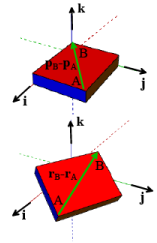When we solve problems, we always express vectors as components in some basis. When we do this, R becomes a matrix. For example, if

PB − PA = x0i + y0j + z0k      rB − rA = xi + yj+zk

we would write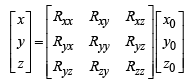Here, R11, R12 , ... are a set of nine numbers (or sometimes formulas).  Following the usual rules of matrixvector multiplication, this is just a short-hand notation for

x = Rxxx0 + Rxyy0 + Rxzz0

y = Ryxx0 + Ryyy0 + Ryzz0

z = Rzxx0 + Rzyy0 + Rzzz0

The subscripts on R are meant to you help remember what each element in the matrix does – for example, Rxx maps the x0 onto x, Rxy maps the y0 onto x, and so on.

So when we solve a problem, how do we go about finding R?  Let me count the ways:

Rotations in two dimensions:

Life is simple in 2D. In this case our rigid body must lie in the i,j plane, so we can only rotate it about an axis parallel to the k direction.  A counterclockwise rotation through an angle θ about the k axis is produced by2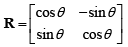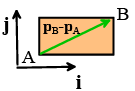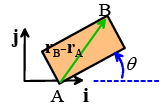For example, a vector Li that start parallel to the i axis is mapped to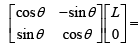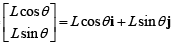3D is a bit more difficult. Any rotation can always be expressed as a rotation through some angle θ about some axis parallel to a unit vector n (we always use the right hand screw convention).  In some problems you can see what n and θ are: then you can write down a unit vector parallel to n

n = nxi +nyj + nzk

and then use the ‘Rodriguez Formula’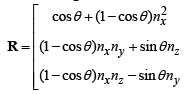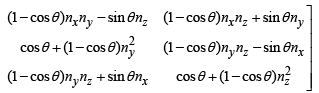(This formula is impossible to remember – that’s what Google is for).

If you are given a rotation matrix R, and need to find n and θ , you can use the formulas:

1 + 2cosθ = Rxx + Ryy + Rzz

n = $${1\over2sinθ}$$ [ (Rzy - Ryz)i +(Rxz - Rzx)j + (Ryx - Rxy)k]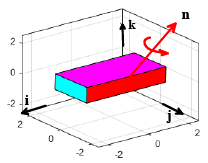The second formula blows up if sin(θ ) = 0 .  If θ is zero or 2π you can simply set R =1 (the identity), and n can be anything you like.

For θ =π you can use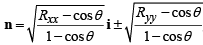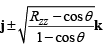The signs of the square roots have to be chosen so that

nxny = Rxy/2 nxnz = Rxz nynz = Ryz /2

In robotics, game engines, and vehicle dynamics the axis-angle representation of a rotation is often stored as a quaternion.  We won’t use that here, but mention it in passing in case you come across it in practice.   A quaternion is four numbers [q0,qx , qy , qx ] that are related to n and θ through the formulas:

qo = cos(θ/2)
q= nx sin(θ/2)    qy = ny sin(θ/2)    qz = nz sin (θ/2)

Mapping the coordinate axes

In some problems we might know what happens to vectors that are parallel to the {i,j,k} directions in the initial rigid body (eg we might know what happens to the sides of our rectangular prism).  For example, we might know that {i, j,k} map to (unit) vectors  a,b, c . In that case we can write down each of a,b, c as components in {i, j,k}

a = axi + ayj+ azk      b = bxi +byj+bzk       c =cxi + cyj+ czk

and use the formula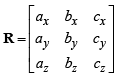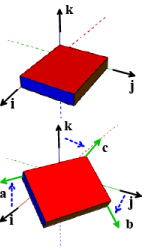A sequence of rotations

Suppose we rotate an object twice (perhaps about two different axes). How do we describe the result of two rotations? That’s not hard. Suppose we do the first rotation with one mapping

rB − rA = R(1) (PB −PA)

Now we rotate our body again – this maps rB −rA onto some new vector uB −uA :

(uB − uA )= R(2)(rB −rA )

We can therefore write

(uB −uA)= R(2) R(1) (pB −pA)

We see that Sequential rotations are matrix products

R =R(2) R(1)

Health warning: Matrix products (and hence sequences of rotations) do not commute

R(1)R(2) ≠ R(2) R(1)

For example, the figure below shows the change in orientation caused by (a) a 90 degree positive rotation about i followed by a 90 degree positive rotation about k (the figure on the left); and (b) a 90 degree positive rotation about k followed by a 90 degree positive rotation about i (the figure on the right).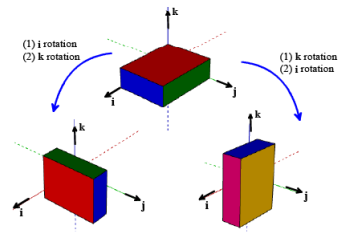Orthogonality of R

The rotation tensor (matrix) has a very important property:

If you multiply R by its transpose, the result is always the identity matrix.

Another way to say this is that

The transpose of R is equal to its inverse

Let’s try this with the 2D rotation matrix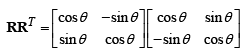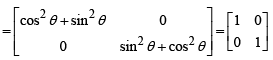A matrix or tensor with this property is said to be orthogonal.

Why is this?  It turns out that a length-preserving mapping must be an orthogonal tensor. To see this, let’s calculate the length of the rotated vector rB − rA = R (PB − PA) .  We need to remember two vector/matrix

operations:

1. We can calculate the length of a vector by dotting it with itself and taking the square root
2. For a vector u and a matrix R, we know (or can show!) that (Ru) ⋅ (Ru)= u ⋅ ( RT Ru )

This means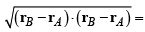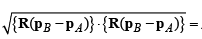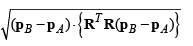But we want the length of rB −rA to equal the length of PB −PA, which means we need R to satisfy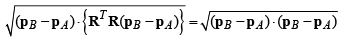⇒ (PB - PA) • {RTR(PB - PA)}- (PB - PA) • {1(PB - PA)} = 0

⇒ (PB - PA) • {(RTR - 1) (PB - PA)} = 0

where 1 is the identity tensor (we normally use I for the identity tensor, but rigid body dynamics uses I to denote the mass moment of inertia so it’s already been taken….). This must hold for all vectors so RR = 1 . In fact, a rigorous mathematical derivation of rotations starts with the statement that R must preserve the length of all vectors, and then derives all the other material in this section from that statement. This is not easy to follow the first time around, but will probably be the approach used in more advanced courses.

Examples:

1. Write down the rotation matrix for the 2D rotation shown in the figure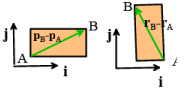The object rotates 90 degree counterclockwise about the k axis, so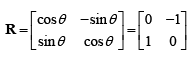2. The object shown in the figure is first rotated 90 degrees about the i axis, and then 180 degrees about the j axis. Find the rotation tensor.

We can construct the two rotations using the Rodriguez formula.  For the first rotation θ =π /2

n =i ⇒ nx= 1 ny = nz =0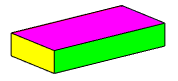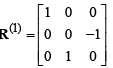For the second rotation θ =π , n =j ⇒ ny = 1 nx =nz = 0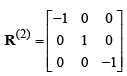The total rotation is therefore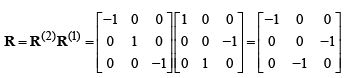3. Find the axis-angle representation for the combined rotation in problem (2).

We can calculate the axis and angle of this rotation using the formulas

1 + 2cosθ = Rxx + Ryy + Rzz ⇒2cosθ= -2 ⇒θ= π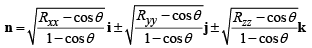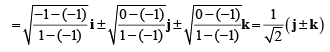To decide which of these two choices to use we notice that Ryz = −1 , which tells us that nynz < 0 .  The answer is therefore

θ =π , n = 1/$${\sqrt{2}}$$ (j - k)

It is incredibly difficult to visualize the effect of a rotation about an arbitrary axis (at least for me). In fact this formula looks wrong – how can a 180 degree rotation end up tipping the box on its side? But the answer is right, as the animation (which will only show up in the html version of the notes) shows.

Offer running on EduRev: Apply code STAYHOME200 to get INR 200 off on our premium plan EduRev Infinity!

## Introduction to Dynamics and Vibrations- Notes, Videos, MCQs

20 videos|53 docs

,

,

,

,

,

,

,

,

,

,

,

,

,

,

,

,

,

,

,

,

,

;